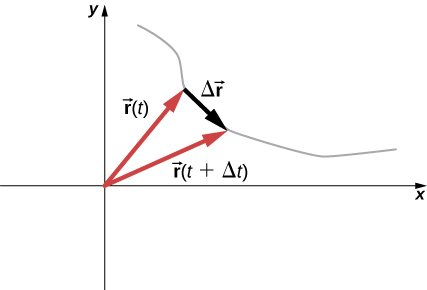# 4.1 Displacement and velocity vectors  (Page 3/7)

 Page 3 / 7A particle moves along a path given by the gray line. In the limit as Δ t approaches zero, the velocity vector becomes tangent to the path of the particle.

[link] can also be written in terms of the components of $\stackrel{\to }{v}\left(t\right).$ Since

$\stackrel{\to }{r}\left(t\right)=x\left(t\right)\stackrel{^}{i}+y\left(t\right)\stackrel{^}{j}+z\left(t\right)\stackrel{^}{k},$

we can write

$\stackrel{\to }{v}\left(t\right)={v}_{x}\left(t\right)\stackrel{^}{i}+{v}_{y}\left(t\right)\stackrel{^}{j}+{v}_{z}\left(t\right)\stackrel{^}{k}$

where

${v}_{x}\left(t\right)=\frac{dx\left(t\right)}{dt},\phantom{\rule{1em}{0ex}}{v}_{y}\left(t\right)=\frac{dy\left(t\right)}{dt},\phantom{\rule{1em}{0ex}}{v}_{z}\left(t\right)=\frac{dz\left(t\right)}{dt}.$

If only the average velocity is of concern, we have the vector equivalent of the one-dimensional average velocity for two and three dimensions:

${\stackrel{\to }{v}}_{\text{avg}}=\frac{\stackrel{\to }{r}\left({t}_{2}\right)-\stackrel{\to }{r}\left({t}_{1}\right)}{{t}_{2}-{t}_{1}}.$

## Calculating the velocity vector

The position function of a particle is $\stackrel{\to }{r}\left(t\right)=2.0{t}^{2}\stackrel{^}{i}+\left(2.0+3.0t\right)\stackrel{^}{j}+5.0t\stackrel{^}{k}\text{m}.$ (a) What is the instantaneous velocity and speed at t = 2.0 s? (b) What is the average velocity between 1.0 s and 3.0 s?

## Solution

Using [link] and [link] , and taking the derivative of the position function with respect to time, we find

(a) $v\left(t\right)=\frac{d\mathbf{\text{r}}\left(t\right)}{dt}=4.0t\stackrel{^}{i}+3.0\stackrel{^}{j}+5.0\stackrel{^}{k}\text{m/s}$

$\stackrel{\to }{v}\left(2.0s\right)=8.0\stackrel{^}{i}+3.0\stackrel{^}{j}+5.0\stackrel{^}{k}\text{m/s}$

Speed $|\stackrel{\to }{v}\left(2.0\phantom{\rule{0.2em}{0ex}}\text{s}\right)|=\sqrt{{8}^{2}+{3}^{2}+{5}^{2}}=9.9\phantom{\rule{0.2em}{0ex}}\text{m/s}.$

$\begin{array}{cc}\hfill {\stackrel{\to }{v}}_{\text{avg}}& =\frac{\stackrel{\to }{r}\left({t}_{2}\right)-\stackrel{\to }{r}\left({t}_{1}\right)}{{t}_{2}-{t}_{1}}=\frac{\stackrel{\to }{r}\left(3.0\phantom{\rule{0.2em}{0ex}}\text{s}\right)-\stackrel{\to }{r}\left(1.0\phantom{\rule{0.2em}{0ex}}\text{s}\right)}{3.0\phantom{\rule{0.2em}{0ex}}\text{s}-1.0\phantom{\rule{0.2em}{0ex}}\text{s}}=\frac{\left(18\stackrel{^}{i}+11\stackrel{^}{j}+15\stackrel{^}{k}\right)\phantom{\rule{0.2em}{0ex}}\text{m}-\left(2\stackrel{^}{i}+5\stackrel{^}{j}+5\stackrel{^}{k}\right)\phantom{\rule{0.2em}{0ex}}\text{m}}{2.0\phantom{\rule{0.2em}{0ex}}\text{s}}\hfill \\ & =\frac{\left(16\stackrel{^}{i}+6\stackrel{^}{j}+10\stackrel{^}{k}\right)\phantom{\rule{0.2em}{0ex}}\text{m}}{2.0\phantom{\rule{0.2em}{0ex}}\text{s}}=8.0\stackrel{^}{i}+3.0\stackrel{^}{j}+5.0\stackrel{^}{k}\text{m/s}.\hfill \end{array}$

## Significance

We see the average velocity is the same as the instantaneous velocity at t = 2.0 s, as a result of the velocity function being linear. This need not be the case in general. In fact, most of the time, instantaneous and average velocities are not the same.

Check Your Understanding The position function of a particle is $\stackrel{\to }{r}\left(t\right)=3.0{t}^{3}\stackrel{^}{i}+4.0\stackrel{^}{j}.$ (a) What is the instantaneous velocity at t = 3 s? (b) Is the average velocity between 2 s and 4 s equal to the instantaneous velocity at t = 3 s?

(a) Taking the derivative with respect to time of the position function, we have $\stackrel{\to }{v}\left(t\right)=9.0{t}^{2}\stackrel{^}{i}\text{and}\stackrel{\to }{v}\text{(3.0s)}=81.0\stackrel{^}{i}\text{m/s}.$ (b) Since the velocity function is nonlinear, we suspect the average velocity is not equal to the instantaneous velocity. We check this and find
${\stackrel{\to }{v}}_{\text{avg}}=\frac{\stackrel{\to }{r}\left({t}_{2}\right)-\stackrel{\to }{r}\left({t}_{1}\right)}{{t}_{2}-{t}_{1}}=\frac{\stackrel{\to }{r}\left(4.0\phantom{\rule{0.2em}{0ex}}\text{s}\right)-\stackrel{\to }{r}\left(2.0\phantom{\rule{0.2em}{0ex}}\text{s}\right)}{4.0\phantom{\rule{0.2em}{0ex}}\text{s}-2.0\phantom{\rule{0.2em}{0ex}}\text{s}}=\frac{\left(144.0\stackrel{^}{i}-36.0\stackrel{^}{i}\right)\phantom{\rule{0.2em}{0ex}}\text{m}}{2.0\phantom{\rule{0.2em}{0ex}}\text{s}}=54.0\stackrel{^}{i}\text{m/s},$
which is different from $\stackrel{\to }{v}\text{(3.0s)}=81.0\stackrel{^}{i}\text{m/s}.$

## The independence of perpendicular motions

When we look at the three-dimensional equations for position and velocity written in unit vector notation, [link] and [link] , we see the components of these equations are separate and unique functions of time that do not depend on one another. Motion along the x direction has no part of its motion along the y and z directions, and similarly for the other two coordinate axes. Thus, the motion of an object in two or three dimensions can be divided into separate, independent motions along the perpendicular axes of the coordinate system in which the motion takes place.

To illustrate this concept with respect to displacement, consider a woman walking from point A to point B in a city with square blocks. The woman taking the path from A to B may walk east for so many blocks and then north (two perpendicular directions) for another set of blocks to arrive at B . How far she walks east is affected only by her motion eastward. Similarly, how far she walks north is affected only by her motion northward.

## Independence of motion

In the kinematic description of motion, we are able to treat the horizontal and vertical components of motion separately. In many cases, motion in the horizontal direction does not affect motion in the vertical direction, and vice versa.

What is angular velocity
refer to how fast an object rotates ot revolve to another point
samson
An object undergoes constant acceleration after starting from rest and then travels 5m in the first seconds .determine how far it will go in the next seconds
x=x0+v0t+1/2at^2
Grant
what is the deference between precision and accuracy
ShAmy
In measurement of a set, accuracy refers to closeness of the measurements to a specific value, while precision refers to the closeness of the measurements to each other.
Grant
Thank you Mr..
ShAmy
Are there is a difference between Error and Relative Error...
ShAmy
An aircraft flies 300km due east and 600km due north. determine the magnitude of its displacement
670.8km
iyiola
which formula did you use
Sophy
X^2=300^2+600^2
iyiola
what is Fermat principle.
find the angle of projection at which the horizontal range is twice the maximum height of a projectile
impulse by height fomula
Hello
what is impulse?
impulse is the integral of a force (F),over the interval for which it act
Agbeyangi
What is significance of vector?
magnitude and direction
Chris
what is impulse?
the product of force and time
Robert
find the flow rate of a fluid of viscosity 0.0015N-s/m³ flowing through a pipe of radius 50cm and length 100m at a pressure differential of 200000N/m²
friend ,what is the equation your question
lasitha
What is victor
How are you all?
what is the formula for momentum?
p=mv
Grant
what is the formula for angular displacement ?
lasitha
angular displacement = l/r
anand
friend ,what is the " l "?
lasitha
I have another problem,what is the difference between pure chemistry and applied chemistry ?
lasitha
l stands for linear displacement and l>>r
anand
r stands for radius or position vector of particle
anand
pure chemistry is related to classical and basic concepts of chemistry while applied Chemistry deals with it's application oriented concepts and procedures.
anand
what is the deference between precision and accuracy?
ShAmy
I think in general both are same , more accuracy means more precise
anand
and lessor error
anand
friends,how to find correct applied chemistry notes?
lasitha
what are the main concepts of applied chemistry ?
lasitha
anand,can you give an example for angular displacement ?
lasitha
when any particle rotates or revolves about something , an axis or point ,then angle traversed is angular displacement
anand
like a revolving fan
anand
yeah ,I understood now, thanks!
lasitha
o need this application in French language
Why is it that the definition is not there
Bello
what is physics
the study of matter in relation to energy
Robert
Ampher law
ZiaByBy